# G.4.4 Practice Problems

Match the equation with a description of the function it represents.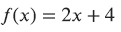Check all that apply

Match the equation with a description of the function it represents.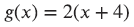Check all that apply

Match the equation with a description of the function it represents.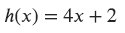Check all that apply

Match the equation with a description of the function it represents.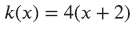Check all that apply

Function P represents the perimeter, in inches, of a square with side length inches. Complete the table below. Write an equation to represent function P.

Sketch a graph of function P.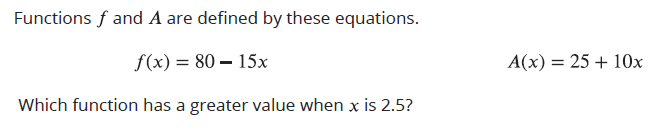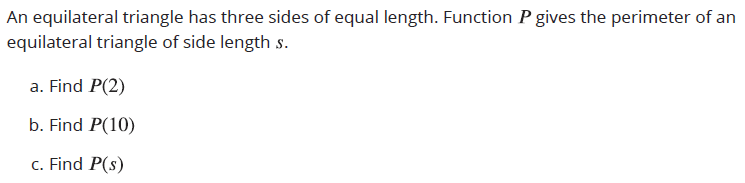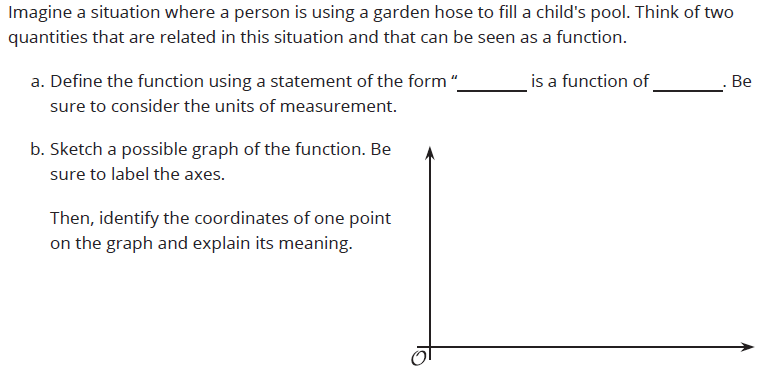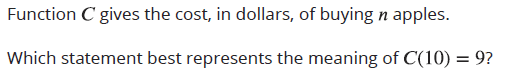Check all that apply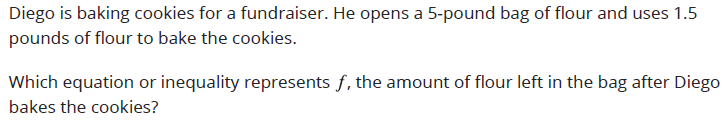Check all that apply